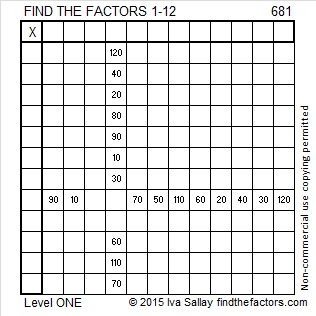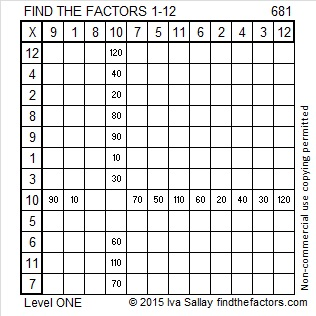# 681 is a factor of the first 681 composite numbers added together

681 can be written as 0681, a number made with three consecutive numbers, 6, 8, and 10 so 681 and every other permutation of 6810 is divisible by 3. The number in the middle of those consecutive numbers is not divisible by 3, so none of those permutations are divisible by 9.

OEIS.org informs us that 681 is a factor of the first 681 composite numbers added together. Here are the number facts and the thought process I used to verify that claim:

• 1 is not a prime number, and it also is not a composite number.
• There are 123 prime numbers less than 681.
• 1 + 123 + 681 = 805, but there are 16 prime numbers between 681 and 805.
• 805 + 16 = 821, but there are 3 prime numbers from 805 to 821.
• 821 + 3 = 824, but there is 1 additional prime number between 821 and 824.
• 824 + 1 = 825 making it the 681st composite number.
• The sum of the numbers from 1 to 825 = (825)(826)/2 = 340,725.
• The sum of all the prime numbers from 2 to 823 is 53,342. (I listed all those prime numbers in excel and summed them.)
• The sum of the first 681 composite numbers is 340,725 – 53,342 – 1 = 287,382.
• (287,382)/681 is exactly 422. Thus 681 is a factor of the first 681 composite numbers added together.

[5(17²) + 5(17) + 2]/2 = 681 making 681 the 17th centered pentagonal number.

Here is a much less mind-boggling factoring puzzle:Print the puzzles or type the solution on this excel file: 12 Factors 2015-11-16

—————————————————————————————————

• 681 is a composite number.
• Prime factorization: 681 = 3 x 227
• The exponents in the prime factorization are 1 and 1. Adding one to each and multiplying we get (1 + 1)(1 + 1) = 2 x 2 = 4. Therefore 681 has exactly 4 factors.
• Factors of 681: 1, 3, 227, 681
• Factor pairs: 681 = 1 x 681 or 3 x 227
• 681 has no square factors that allow its square root to be simplified. √681 ≈ 26.0959767.—————————————————————————————————This site uses Akismet to reduce spam. Learn how your comment data is processed.